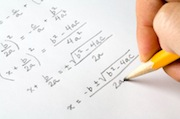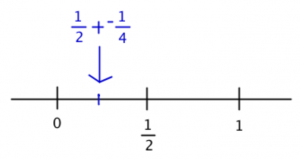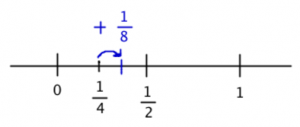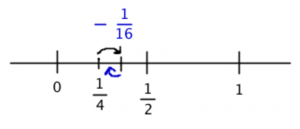Want a 51 on Quant? Answer This Problem!:

by on January 24th, 2014Sequence problems aren’t incredibly common on the test, but if you’re doing well on the quant section, be prepared to see one. Now, you’ve got a choice: do you want to guess quickly and save time for other, easier topics? Do you want to learn some “test savvy” techniques that will help you with some sequence questions but possibly not all of them? Or do you want to learn how to do these every single time, no matter what?

That isn’t a trick question. Every good business person knows that there’s a point of diminishing returns: if you don’t actually need a 51, then you may study for a lower (but still good!) score and re-allocate your valuable time elsewhere.

Try this GMATPrep® problem from the free test. After, we’ll talk about how to do it in the “textbook” way and in the “back of the envelope” way.

* ”For every integer k from 1 to 10, inclusive, the kth term of a certain sequence is given by. If T is the sum of the first 10 terms in the sequence, then T is

“(A) greater than 2

“(B) between 1 and 2

“(C) betweenand 1

“(D) betweenand“(E) less thanFirst, let’s talk about how to do this thing in the “textbook math” way. If you don’t want to do this the textbook math way, feel free to skip to the second method below.

Textbook Method

If you’ve really studied sequences, then you may recognize the sequence as a particular kind called a Geometric Progression. If not, you would start to find the terms and see whether you can spot a pattern.

Plug in k = 1, 2, 3. What’s going on?

If k = 1, thenIf k = 2, thenIf k = 3, thenWhat’s going on here? Each time, the term gets multiplied byin order to get to the next one. When you keep multiplying by the same number in order to get to the next term, then you have a geometric progression.

This next part gets into some serious math. Unless you really just love math, I wouldn’t bother learning this part for the GMAT, because there’s a very good chance you’ll never need to use it. But, if you want to, go for it!

When you have a geometric progression, you can calculate the sum in the following way:Next, you’re going to multiply every term in the sum by the common ratio. What’s the common ratio? It’s the constant number that you keep multiplying each term by to get the next one. In this case, you’ve already figured this out: it’s.

If you multiply this through all of the terms on both sides of the equation, you’ll get this:Does anything look familiar? It’s basically the same list of numbers as in the first sum equation, except it’s missing the first number,. All of the others are identical!

Subtract this second equation from the first:The right-hand side of the equation is always going to be just the first term of the original sum. The rest of the terms on the right-hand side of the two equations are identical, so when you subtract, they become zero and disappear.

Solve for s:This value falls between 1/4 and 1/2, so the answer is (D).

Back of the Envelope Method

There is another way to tackle this one. At the same time, this problem is really tricky—so this solution is still not an “easy” solution. Your best choice might be just to guess and move on.

Before you start reading the text, take a First Glance at the whole thing. It’s a problem-solving problem. The answers are… weird. They’re not exact. What does that mean?

Read the problem, but keep that answer weirdness in mind. The first sentence has a crazy sequence. The question asks you to sum up the first 10 terms of this sequence. And the answers aren’t exact… so apparently you don’t need to find the exact sum.

Take a closer look at the form of the answers. Notice anything about them?

They don’t overlap! They cover adjacent ranges. If you can figure out that, for example, the sum is about 3/4, then you know the answer must be (C). In other words, you can actually estimate here—you don’t have to do an exact calculation.

That completely changes the way you can approach this problem! Here’s the sequence:According to the problem, the 10 terms are from k = 1 to k = 10. Calculating all 10 of those and then adding them up is way too much work (another clue that there’s got to be a better way to do this one). So what is that better way?

Since you know you can estimate, try to find a pattern. Calculate the first two terms (we had to do this in the first solution, too).

If k = 1, thenIf k = 2, thenWhat’s going on? The first answer is positive and the second one is negative. Why? Ah, because the first part of the calculation is -1 raised to a power. That will just keep switching back and forth between 1 and -1, depending on whether the power is odd or even. It won’t change the size of the final answer, but it will change the sign.

Okay, and what about that second part? it went from 1/2 to 1/4. What will happen next time? Try just that part of the calculation. If k = 3, then just that part will become.

Interesting! So the denominator will keep increasing by a factor of 2: 2, 4, 8, 16 and so on.

Great, now you can write out the 10 numbers!… ugh. The denominator’s getting pretty big. That means the fraction itself is getting pretty small. Do I need to keep writing these out?

What was the problem asking again?

Right, find the sum of these 10 numbers. Let’s see. The first number in the sequence is 1/2 and the second is -1/4, so the pair adds up to 1/4.Right now, the answer would be right between D and E. Does the sum go up or down from here?

The third number will add 1/8, so it goes up:But the fourth will subtract 1/16 (don’t forget that every other term is negative!), pulling it back down again:Hmm. In the third step, it went up but not enough to get all the way to 1/2. Then, it went down again, but by an even smaller amount, so it didn’t get all the way back down to 1/4.

The fifth step would go up by an even smaller amount (1/32), and then it would go back down again by yet a smaller number (1/64). What can you conclude?

First, the sum is always growing a little bit, because each positive number is a bit bigger than the following negative number. The sum is never going to drop below 1/4, so cross off answer (E).

You keep adding smaller and smaller amounts, though, so if the first jump of 1/8 wasn’t enough to get you up to 1/2, then none of the later, smaller jumps will get you there either, especially because you also keep subtracting small amounts. You’re never going to cross over to 1/2, so the sum has to be between 1/4 and 1/2.

As I mentioned above, you may decide that you don’t want to do this problem at all. These aren’t that common—many people won’t see one like this on the test. Also, you don’t have to get everything right to get a top score. Just last week, I spoke with a student who outright guessed on 4 quant problems, and she still scored a 51 (the top score).

Key Takeaways for Advanced Sequence Problems

(1) Do you even want to learn how to do these? Don’t listen to your pride. Listen to your practical side. This might not be the best use of your time.

(2) All of these math problems do have a textbook solution method—but you’d have to learn a lot of math that you might never use if you try to learn all of the textbook methods. That’s not a problem if you’re great at math and have a great memory for this stuff. If not…

(3) … then think about alternate methods that can work just as well. Certain clues will indicate when you can estimate on a problem, rather than solving for the “real” number. You may already be familiar with some of these, for instance when you see the word “approximately” in the problem or answer choices that are spread pretty far apart. Now, you’ve got a new clue to add to your list: answers that offer a range of numbers and the different answer ranges don’t overlap.

* GMATPrep® questions courtesy of the Graduate Management Admissions Council. Usage of this question does not imply endorsement by GMAC.

•I started going through the explanation and realised half way through that such detailed explanation can be given only by stacey and yes i was right!!!
Stacey you are the bestttt!!!

•HI stacey
you have posted a sequence gmat prep problem on 24th jan
can we do this by formula of sum of terms of geometric progression in 1 min

it will be
1/2(1-(-1/2)ˆ10)/(1-(-1/2)

1/3(1-1/1024)

since 1/1024 is very small as compared to 1 we can ignore it

ans 1/3

correct option D

•Yes ishaan, even i did it the same way. So i guess its pretty much ok.•The geometric series is: 1/2 - 1/4 + 1/8 - 1/16+1/32-1/64+.....
Rearranged as: 1/2 - (1/4 - 1/8) - (1/16-1/32) - ....

Differences of fractions in brackets (i.e. (1/4 - 1/8) and subsequent ones) are all positive.
So, : 1/2 - (something positive)
Hence the sum of the series is less than 1/2.

Again this something positive is 1/4 - 1/8 + 1/16 - 1/32 + 1/64-....., which is again by above logic: 1/4 - (some other positive no.) and hence less than 1/4

So sum of original series is: 1/2 - (something less than 1/4 but positive). Between 1/4 and 1/2. Hence option D.